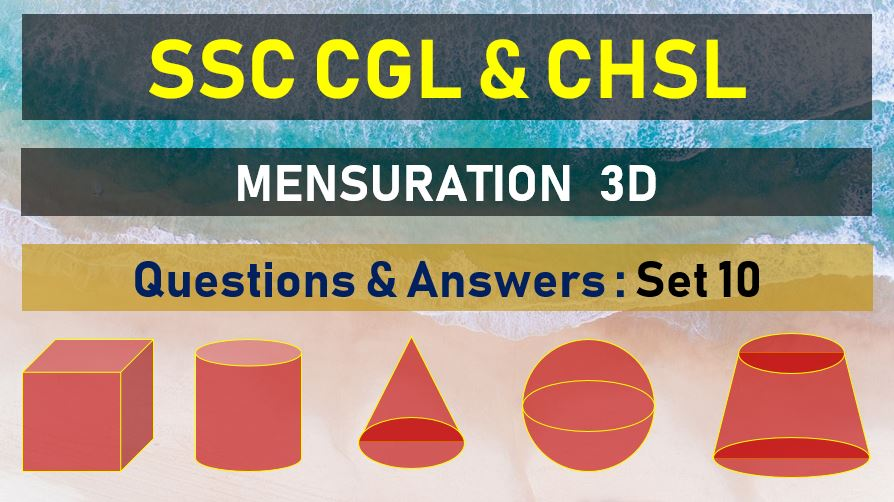Tuesday, June 22, 2021
Home > mensuration > SSC CHSL Surface Area and Volume Questions Answers Set 10

# SSC CHSL Surface Area and Volume Questions Answers Set 10SSC Mensurations 3D
Surface Area & Volume Questions Sets
Set 1Set 2Set 3Set 4Set 5
Set 6Set 7Set 8Set 9Set 10

SSC CHSL Surface Area and Volume Questions Answers Set 10: Ques No 1

The volumes of a right circular cylinder and a sphere are equal. The radius of the cylinder and the diameter of the sphere are equal, The ratio of height and radius of the cylinder is

Options:

A. 3 : 1

B. 1 : 3

C. 6 : 1

D. 1 : 6

Answer: D

Solution:

SSC CHSL Surface Area and Volume Questions Answers Set 10: Ques No 2

If the ratio of volumes of two cones is 2 : 3 and the ratio of the radii of their bases is 1 : 2, then the ratio of their heights will be

Options:

A. 8 : 3

B. 3 : 8

C. 4 : 3

D. 3 : 4

Answer: A

Solution:

SSC CHSL Surface Area and Volume Questions Answers Set 10: Ques No 3

The total number of spherical bullets each of diameter 5 decimeter that can be made by utilizing the maximum of a rectangular block of lead with 11 meter length, 10 meter breadth and 5 meter is (assume that π =3 )

Options:

A. 8800

B. 8000

C. 7800

D. 7790

Answer: A

Solution:

SSC CHSL Surface Area and Volume Questions Answers Set 10: Ques No 4

The ratio of weights of two spheres ratio of different materials is 8 : 17 and the ratio of weights per 1 cc of materials of each is 289 : 64. The ratio of radii of the two spheres is

Options:

A. 8 : 17

B. 4 : 17

C. 17 : 4

D. 17 : 8

Answer: A

Solution:

SSC CHSL Surface Area and Volume Questions Answers Set 10: Ques No 5

Two cubes have their volumes in the ratio 27 : 64. The ratio of their surface areas is

Options:

A. 9 : 25

B. 16 : 25

C. 9 : 16

D. 4 : 9

Answer: C

Solution:

SSC CHSL Surface Area and Volume Questions Answer Set 10: Ques No 6

A solid sphere is melted and recast into a right circular cone with a base radius equal to the radius of sphere. What is the ratio of the height and radius of the cone so formed

Options:

A. 4 : 3

B. 2 : 3

C. 3 : 4

D. 4 : 1

Answer: D

Solution:

SSC CHSL Surface Area and Volume Questions Answer Set 10: Ques No 7

The volume of a cylinder and a cone are in the ratio 3 : 1. Find their diameters and then compare them when their height is equal

Options:

A. diameter of cylinder = 2 times of diameter of cone

B. diameter of cylinder = diameter of cone

C. diameter of cylinder > diameter of cone

D. diameter of cylinder < diameter of cone

Answer: B

Solution:

SSC CHSL Surface Area and Volume Questions Answer Set 10: Ques No 8

A sphere and a hemisphere have the same volume. The ratio of their curved surface area is

Options:

A. 23/2: 2

B. 22/3: 2

C. 42/3: 1

D. 21/3: 1

Answer: D

Solution:

SSC CHSL Surface Area and Volume Questions Answer Set 10: Ques No 9

Diagonal of a cube is 6√3 cm. ratio of its total surface area and volume (numerically) is

Options:

A. 2 : 1

B. 1 : 6

C. 1 : 1

D. 1 : 2

Answer: C

Solution:

SSC CHSL Surface Area and Volume Questions Answer Set 10: Ques No 10

The radii of the base of cylinder and cone are in ratio √3: √2 and their heights are in the ratio √2: √3 . Their volumes are in the ratio of

Options:

A. √3: √2

B. 3√3: √2

C. √3:2√2

D. √2:√6

Answer: B

Solution:

SSC CHSL Surface Area and Volume Questions Answer Set 10: Ques No 11

A hall is 15 m long and 12 m broad. If the sum of the areas of the floor and the ceiling is equal to the sum of the areas of four walls, the volume of the hall is:

Options:

A. 720

B. 900

C. 1200

D. 1800

Answer: C

SSC Mensurations 3D
Surface Area & Volume Questions Sets
Set 1Set 2Set 3Set 4Set 5
Set 6Set 7Set 8Set 9Set 10
error: Content is protected !!